Chapter 21 Electric Charge and electric Field Charles

• Slides: 9Chapter 21, Electric Charge, and electric Field Charles Allison © 2000Problem 12 12. (II) Particles of charge +75 μC, +48μC, and -85μC are placed in a line (Fig. 21– 52). The center one is 0. 35 m from each of the others. Calculate the net force on each charge due to the other two. Charles Allison © 200021 -5 Coulomb’s Law Example 21 -3: Electric force using vector components. Calculate the net electrostatic force on charge Q 3 shown in the figure due to the charges Q 1 and Q 2. Charles Allison © 2000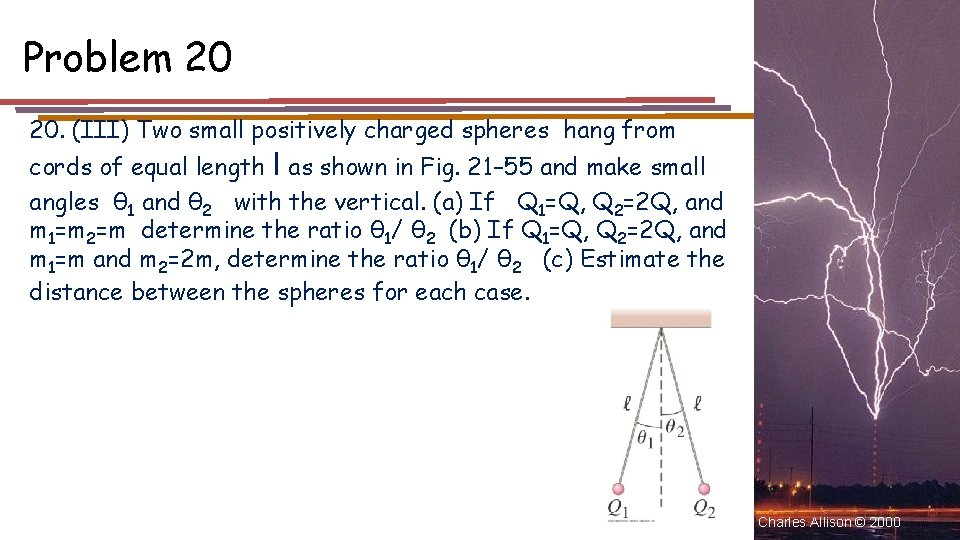Problem 20 20. (III) Two small positively charged spheres hang from cords of equal length l as shown in Fig. 21– 55 and make small angles θ 1 and θ 2 with the vertical. (a) If Q 1=Q, Q 2=2 Q, and m 1=m 2=m determine the ratio θ 1/ θ 2 (b) If Q 1=Q, Q 2=2 Q, and m 1=m and m 2=2 m, determine the ratio θ 1/ θ 2 (c) Estimate the distance between the spheres for each case. Charles Allison © 2000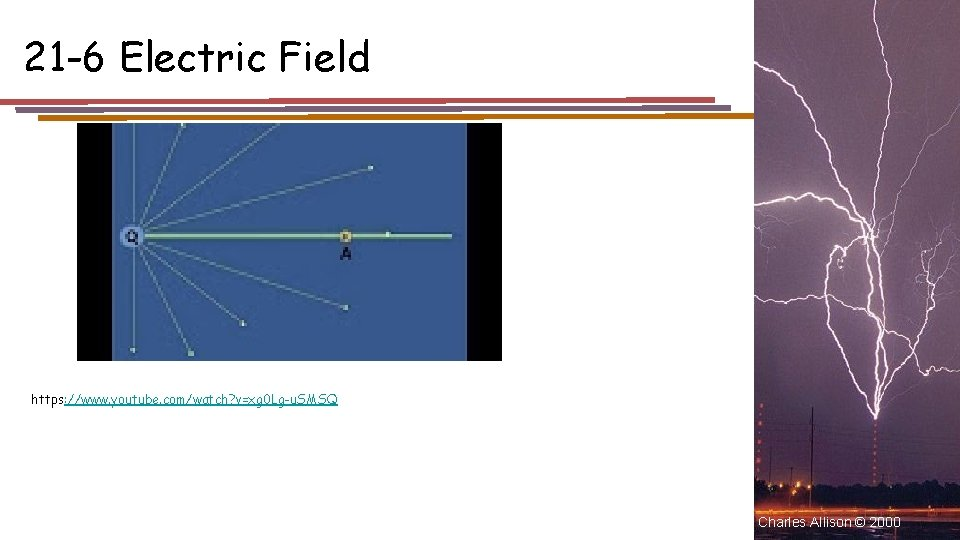21 -6 Electric Field https: //www. youtube. com/watch? v=xg 0 Lg-u. SMSQ Charles Allison © 2000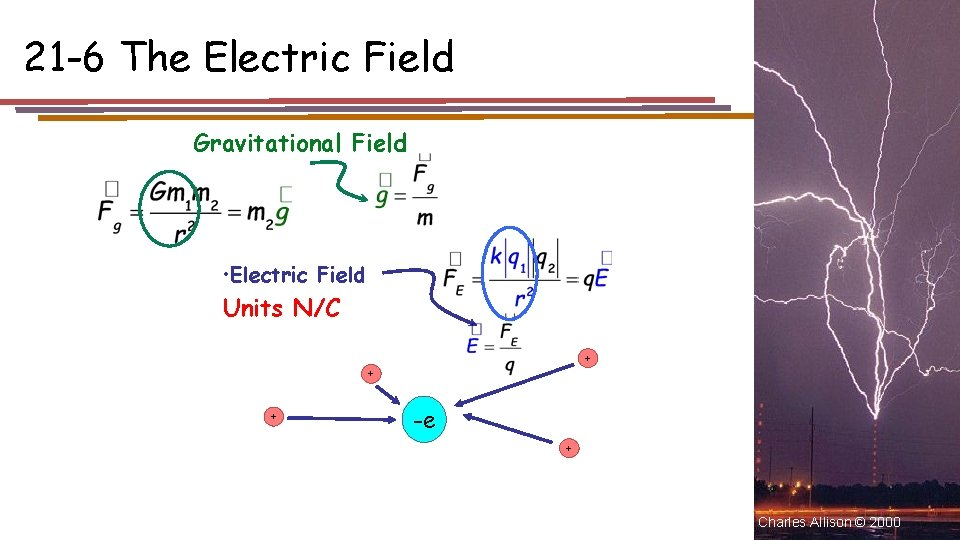21 -6 The Electric Field Gravitational Field • Electric Field Units N/C + + + -e + Charles Allison © 2000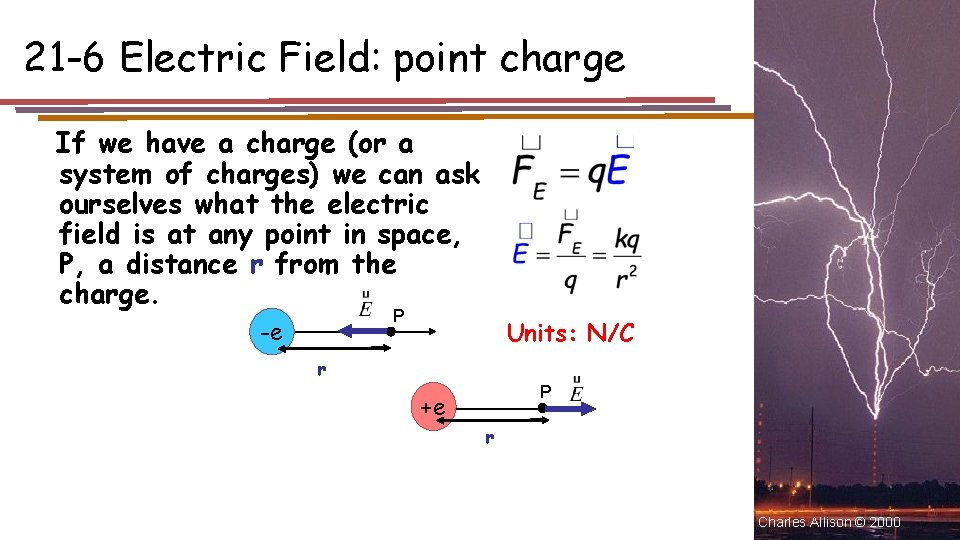21 -6 Electric Field: point charge If we have a charge (or a system of charges) we can ask ourselves what the electric field is at any point in space, P, a distance r from the charge. P -e Units: N/C r P +e r Charles Allison © 200021 -6 The Electric Field The electric field is defined as the force on a small charge, divided by the magnitude of the charge: The electric field is a vector Charles Allison © 2000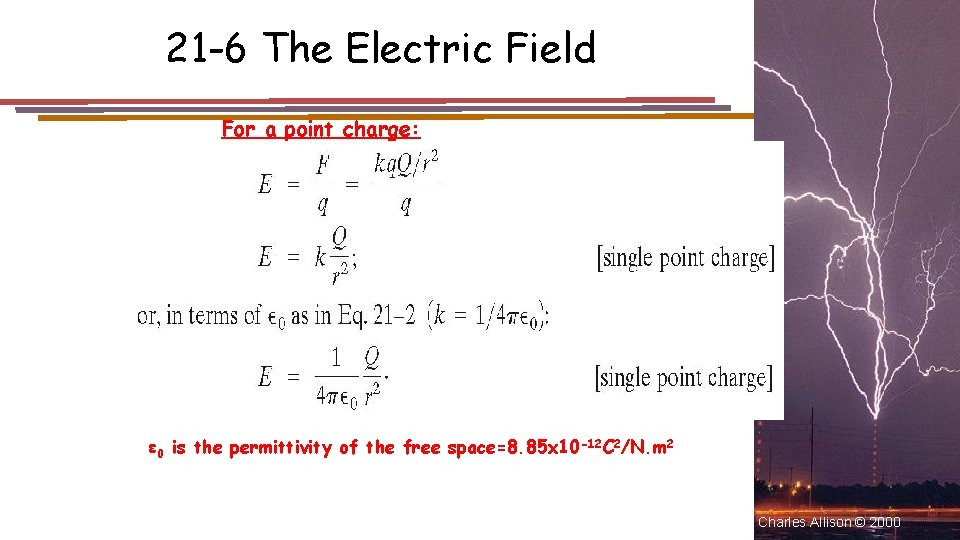21 -6 The Electric Field For a point charge: ε 0 is the permittivity of the free space=8. 85 x 10 -12 C 2/N. m 2 Charles Allison © 2000